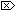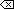The Open Group Base Specifications Issue 6
IEEE Std 1003.1-2001

#### NAME

remainder, remainderf, remainderl - remainder function

#### SYNOPSIS

```#include <math.h> double remainder(double x, double y); float remainderf(float x, float y); long double remainderl(long double x, long double y); ```

#### DESCRIPTION

[CX]The functionality described on this reference page is aligned with the ISO C standard. Any conflict between the requirements described here and the ISO C standard is unintentional. This volume of IEEE Std 1003.1-2001 defers to the ISO C standard.These functions shall return the floating-point remainder r= x- ny when y is non-zero. The value n is the integral value nearest the exact value x/ y. When |n-x/y|=½, the value n is chosen to be even.

The behavior of remainder() shall be independent of the rounding mode.

#### RETURN VALUE

Upon successful completion, these functions shall return the floating-point remainder r= x- ny when y is non-zero.

[MX]If x or y is NaN, a NaN shall be returned.

If x is infinite or y is 0 and the other is non-NaN, a domain error shall occur, and either a NaN (if supported), or an implementation-defined value shall be returned.#### ERRORS

These functions shall fail if:

Domain Error
[MX]The x argument is ±Inf, or the y argument is ±0 and the other argument is non-NaN.

If the integer expression (math_errhandling & MATH_ERRNO) is non-zero, then errno shall be set to [EDOM]. If the integer expression (math_errhandling & MATH_ERREXCEPT) is non-zero, then the invalid floating-point exception shall be raised.The following sections are informative.

None.

#### APPLICATION USAGE

On error, the expressions (math_errhandling & MATH_ERRNO) and (math_errhandling & MATH_ERREXCEPT) are independent of each other, but at least one of them must be non-zero.

None.

#### FUTURE DIRECTIONS

None.

abs() , div() , feclearexcept() , fetestexcept() , ldiv() , the Base Definitions volume of IEEE Std 1003.1-2001, Section 4.18, Treatment of Error Conditions for Mathematical Functions, <math.h>

#### CHANGE HISTORY

First released in Issue 4, Version 2.

#### Issue 5

Moved from X/OPEN UNIX extension to BASE.

#### Issue 6

The remainder() function is no longer marked as an extension.

The remainderf() and remainderl() functions are added for alignment with the ISO/IEC 9899:1999 standard.

The DESCRIPTION, RETURN VALUE, ERRORS, and APPLICATION USAGE sections are revised to align with the ISO/IEC 9899:1999 standard.

IEC 60559:1989 standard floating-point extensions over the ISO/IEC 9899:1999 standard are marked.

End of informative text.

UNIX ® is a registered Trademark of The Open Group.
POSIX ® is a registered Trademark of The IEEE.
[ Main Index | XBD | XCU | XSH | XRAT ]